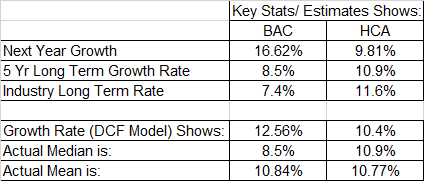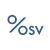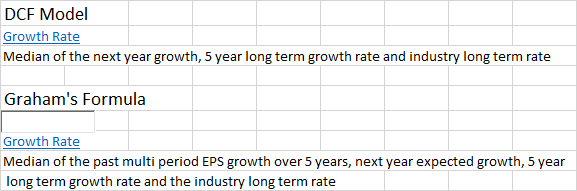0
Fixed

# DCF Growth Rate

Jeff Partlow 6 years ago updated by OSV Support 6 years ago

The default Growth Rate % used in DCF Valuation model seems incorrect based on the description of how it is determined (i.e. median of 3 growth estimates). Two examples (BAC and HCA) showing this:Related question: Should the default Graham model Growth Rate % be different from that used by DCF? That is, for Graham is it necessary to add the 4th variable (past 5 years)? Using BAC for example, adding the 4th variable apparently catapults the Growth Rate from 8.5% (median based on 3 variables in the DCF model) to 16.62% shown for the Graham model. +16.62% is an exceeding high number that defies common sense for BAC's future growth prospects.Another example I was just looking at:

In 'Step 3 -- Valuation' models, 'Growth Rate' for GM is +5.21% for DCF model and +9.42% for Graham's Formula model. As with BAC example above, DCF model 'Growth Rate' seems more realistic.We don't use the same growth rate for each model because DCF is still focused on cash flows, whereas Graham doesn't factor in cash flow growth rates.I disagree with using different company future growth rate estimates for two models (DCF and Graham), but that's your prerogative. As an example, Bank of America is a very large, slow growth company. The Growth Rate assumptions shown above of 12.56% for DCF and 16.62% for Graham are (1) Too high for this slow future growth company; and (2) a growth rate variance of 4.06% (16.62% - 12.56%) is much too high -- and fails the common sense test.

However, that is not really the main point in the initial post. The main point is that the 'Growth Rate' %s shown as the default numbers for both the DCF model and the Graham's Formula model are incorrect when calculating using the respective 'Growth Rate' bubble definitions provided in the app, which are shown to be:As shown in the original post for Bank of America (BAC), if the DCF Model definition is used, the actual median 'Growth Rate' % would be 8.5%, not the 12.56% shown in the app. For both models, either (1) there is a calculation error in the app; or (2) the formula being used is different from the definitions as shown in the app for both models.Fixed

Updated tooltip text.